# Creating a balanced multi-label dataset for machine learning

Teaching a machine to categorize something into multiple, non-exclusive groups can feel a little lot juggling. You’ve got to keep track of a lot of things and if any one thing gets out of balance, everything can come crashing down.

Here at GumGum, we use machine learning and AI to analyze images and text to make sure we don’t place ads on web pages with brand unsafe or threatening content (to learn more about our brand safety models at GumGum, check out this video and this blog post). That means we’re constantly experimenting, and we’re constantly playing with state-of-the-art deep learning models and techniques, in order to deploy high performance models, models which process literally millions of web pages a day. In this blog post, I want to discuss one of the challenges that we encounter when dealing with imbalanced data in a multi-label classification problem and then present a novel technique that we’ve started using to create more balanced training datasets, while also lowering annotation costs.

First, let’s talk a bit about what exactly multi-label classification is and some problems that are likely to arise when trying to train a machine learning model. Multi-label classification is when you try to predict certain properties, or labels, for each item in the data. For instance, does this image have a dog in it, a cat in it or a hat in it? Unlike multi-class classification, where each item has exactly one class, each can have any number of labels. Continuing the example above, an image can have the “dog” and “hat” labels, or just “dog”, or just “hat” or neither and the same goes for all other permutations of the labels in the dataset.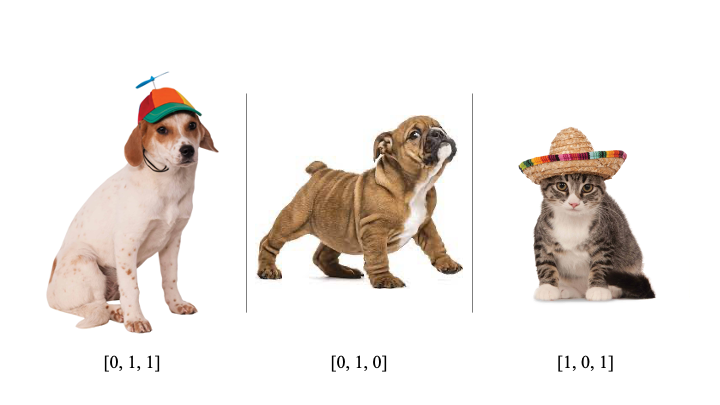Data examples for a very important problem: does the image include a cat, a dog or a hat? Some images match more labels than one.

This means that you can run into problems if there’s a particular label that appears much more or much less often in the dataset than others. Machine learning models are notorious for exaggerating distributional biases in the data they’re trained on, meaning that if a label is rare in the training data, the model may have trouble identifying examples when its making predictions in the real world. Similarly, if a label is very common in the training data, the model may begin to predict that label for nearly every example it sees.

This is a common problem in machine learning broadly and, as such, there are a lot of clever ways to mitigate the problem of label imbalance, such as over- or under-sampling — taking more or fewer of one label or another when training — or generating new synthetic images, text or tabular examples based on patterns already in the data (check out this Python library for some handy implementations). Yet, these techniques are mainly focused on generating examples for multi-class problems, where each item has only a single label. Label imbalance in a multi-label dataset, on the other hand, can be harder to solve than simply implementing one of these off-the-shelf algorithms. Now, a little label imbalance is not bad, per se. To train a performant model, you may still want to contain some distributional biases in your data, e.g., you might want more pictures of dogs without hats compared to those with hats since dogs generally prefer to be hatless. In that case, there still are some techniques, albeit a little more involved, to effectively split up multi-label datasets.

But, what about cases when you want a dataset where each of the labels in a multi-label problem is equally represented? For example, a balanced validation set can be useful in exploring what about the input causes the model to predict one label or another. A balanced dataset can also be a useful starting point to augment an existing training set with new examples in an active learning pipeline. Because the kinds of things on web pages and the internet as a whole is constantly changing, we on the CV and NLP team are regularly collecting and annotating training data to allow them to catch new trends on the web and to improve performance overall. This presents us with a problem: which web pages do we select to be annotated and added to our training data?

As you might’ve guessed, there are a lot of web pages out there and if we were to pick pages blindly, we would not end up with a balanced dataset. In fact, we would likely end up with data that do not help our models learn much, but still cost the time and money to annotate. Considering this, we have a distinct need to smartly select web pages from the billions out there and create a balanced set, which we can then annotate and add to our training dataset. A strategy that we’ve begun to use involves using our existing ML models to select web pages from the millions we analyze every day that are likely to be examples of one or more of our threat classes and combine them into a large imbalanced dataset. We then use the technique described below to create a balanced dataset, which we pass on to human annotators. This ensures both a good number of examples for less frequent labels and lets us more quickly and cost-effectively collect and annotate training data for our models.

To show the technique behind how we create a balanced multi-label dataset, I’ll use a public dataset as an example. I’m a linguist by trade, so I’ll use a natural language example here, though this technique is agnostic of the type of data and can work for any type of input. We’ll start with the toxic comments dataset, which is a multi-label dataset of comments collected from Wikipedia’s talk pages. I like this set in particular as an example because the task is similar to identifying unsafe or threatening content on web pages and there’s an interesting set of relationships between the labels and a high level of collinearity between labels (I’ll touch more on that soon). First, let’s take a quick look at the data themselves.

Each item in the dataset is a string of text, with one of 6 labels which cover various kinds of threatening content or toxicity in the text. As this is a multi-label dataset, each text string can have 0–6 labels associated to it, with 0 labels being non-toxic. Here’s a plot of the number of labels per item.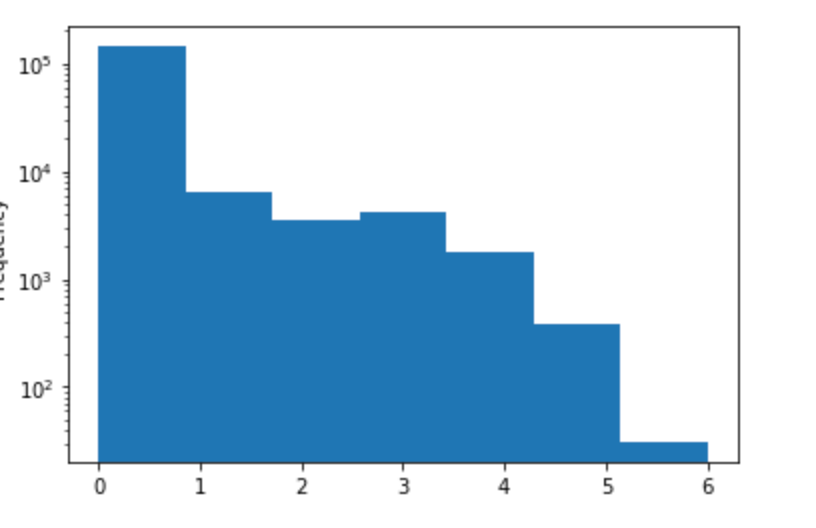Number of toxic labels per item in the toxic comment dataset.

As you can see, nearly as many items have two or three toxic labels as have just one. Now, here’s a quick plot of the distribution of each label, that is, how many items in total show a particular kind of toxic language.

Now, here is where it gets interesting. As you can see, there’s a clear imbalance between the kinds of toxic language used in the dataset. Overall, the labels show a Zipfian distribution (I’m a linguist, I had to mention Zipf somehow), with some labels occurring several times more than the other labels. Now, what does this mean for us? First, the good news. The good news is that highly imbalanced data like we see here is actually common in practice and the used methods to mitigate these effects will work for other multi-label problems out there. Now, it’s time for the bad news. The bad news is that if we were to blindly take a random percentage of the dataset — like is commonly done to split up data into train and validation sets — we would create a new set that still has a large discrepancy between the most and least frequent labels and, perhaps, even misses some of the least frequent labels altogether.

Of course, randomly taking a percentage is not the only thing we can do. If we want a new dataset with an equal number of examples per label, we could try a naive sampling strategy where we loop through the dataset and select a set number of examples that match the current label. That is, we filter out every comment that does not match `toxic` and take some of those, then filter out everything that does not match `insult` and take some of those and so on. If we take N examples per label, that will give us a new set where we have N examples minimum per label, which is on the right track. However, doing this, we rather end up with a sampled set that is still quite imbalanced.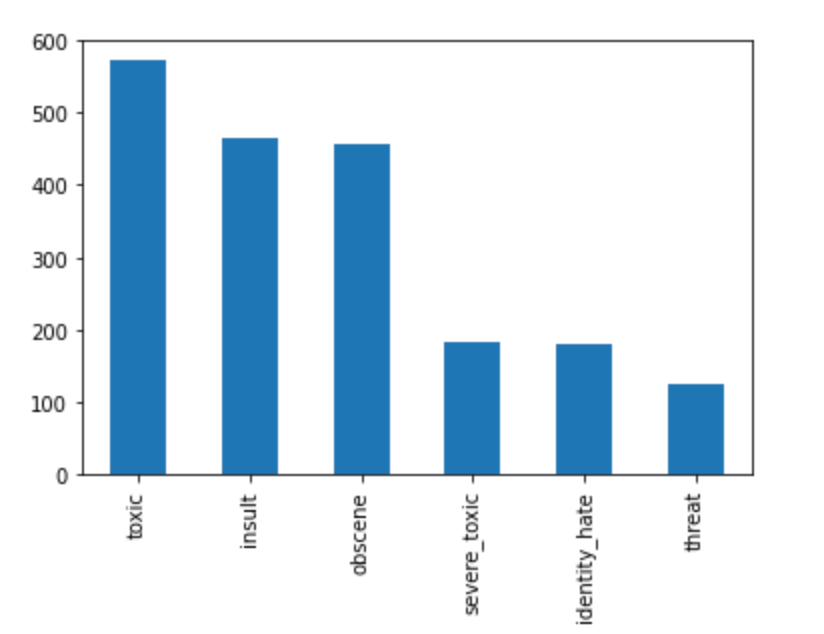Label distribution by randomly selecting N = 100 examples for each of the 6 toxic categories.

What’s going on here? Granted, we’ve definitely reduced the discrepancy between the most common labels and the least common ones, but we’re still left with a very imbalanced set. If this were a multi-class dataset, this would have been the an ideal solution and we would have exactly N examples per label. To understand why this sampling method didn’t work here, recall that as many items in the dataset have two or three labels as have one. This means that when we select an item based on matching one label, we’re very often picking an example of another label by chance.

To see how this affects us, let’s look a little closer at the data, in particular looking at the label `severe_toxic`. If we filter the dataset to only comments that have the label `severe_toxic` and look at the breakdown of labels, a striking patten emerges.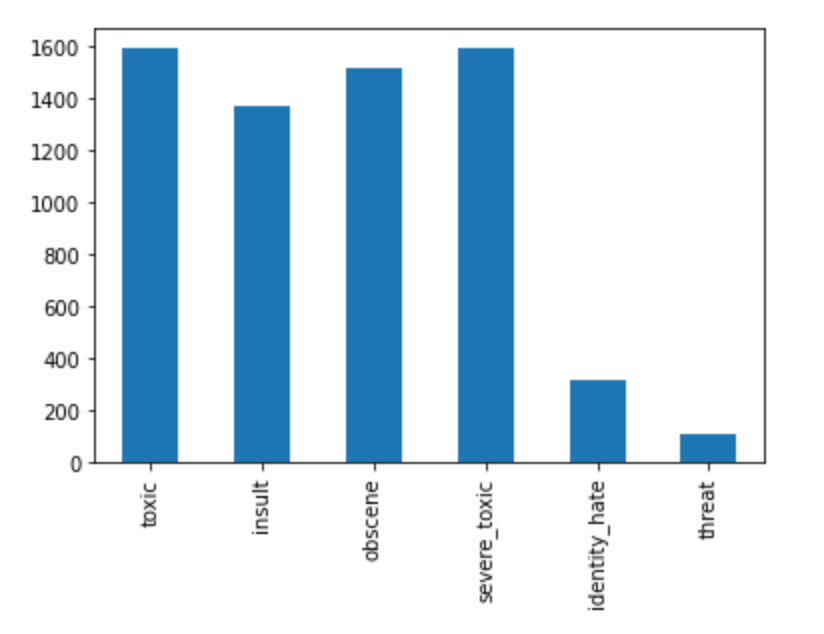Label distribution for examples in the toxic comment dataset that match the label “severe_toxic”.

Notice that every example of `severe_toxic` is also an example of `toxic`, and many are `obscene` or `insult` as well. Moreover, the frequency of co-occurence is not simply a function of frequency in the dataset as whole; `obscene` is more frequent than `insult` here, even though `insult` is more common across the whole dataset. What this means is that some labels are more correlated with others, and as a product of this, there is in fact a pattern of exactly which labels we’re grabbing by chance when we sample `severe_toxic` examples.

I think it’s helpful if we expand this view into the data and look at an overall correlation plot. This plot shows us how likely two labels are to co-occur in a dataset, i.e., the probability of one label occurring for the same input as another. As you’d expect, the diagonal is all 1’s, since every label always co-occurs with itself. Looking beyond the diagonals, we see many values that are greater than .5, meaning that some labels occur together more often than not.

What does that mean for us? Well, it means that when we were doing our naive sampling earlier and grabbing examples of `severe_toxic` , we were always grabbing examples of `toxic` as well and often other labels on top of that. It is precisely because of the correlation between these two labels that our naive sampling method failed to give us a balanced set. It seems that we have run into a bit of a snag if we want to collect a balanced set.

Now, it’s time for the solution. Let’s step back from this and talk about what we’re trying to do at a high level. This will include a little mathematical notation for clarity, but the problem is relatively simple and straightforward.

What we want is the final count for each label, `C_i`, e.g. `C_toxic`, in our sampled dataset to all be equal to the same value, which I will denote with a fancy ℂ. As we’ve seen, the count in for an individual label the final dataset is actually the sum of a) the times we select for that label specifically and b) the times we select for a different label which happens to also match the label in question.

Let’s denote the number of times we sample from the dataset for a specific label as `c_i` where i is the label, e.g., `c_toxic`. Note for the sake of formalism, `C_toxic` and `c_toxic` are distinct but related values. `C_toxic` is the number of all examples that have the `toxic` label after we’ve sampled for each and every label, while `c_toxic` is the times we sample for `toxic` specifically. With this in mind, let’s represent the probability that we get an example of label `i` when sampling for another label `j`, as `p(i|j)`, e.g., `p(toxic|severe)` is the probability of `toxic` when we sample for `severe`. What this means for us is that:

And the same goes for all other labels. Now, let’s talk a little bit about `p(i|j)`. Earlier, I showed a plot of the Pearson’s correlation between the labels in the original distribution, which is a symmetric relation between the two labels. What we want is the conditional probabilities, which show how likely one label is, if we already know another label, which is not symmetric. Luckily, this can be estimated using the original data. It’s trivial to calculate the joint probability of each pair of labels, `p(i,j)`and then we can use the chain rule of probability, to calculate `p(i|j) = p(i,j) / p(j)`. At this point, it’s important to point out, that this is a simplification of the true multi-collinearity of the dataset — it only takes one other label’s correlation with the target into account — but this simplification makes the problem much more tractable than using a six-dimensional correlation tensor and I’ll use it because of that.

The below table shows the simplified conditional probabilities of each label: given the label denoted by the column, the chance an item in the dataset also matches the label denoted by the row. Recall that every example of `severe_toxic` was also an example of `toxic`? You can see that this is represented here, since `p(toxic|severe)`= 1 (row 1, column 2 in the matrix below).

Expanding from this relationship, we can take the values from the matrix and fill in values in the equation earlier.

This is pretty cool. The only variables left are the counts per label and what we’ve ended up with is a simple system of linear equations. We can now use a simple, tried-and-true form of linear regression, non-negative least square regression (non-negative because we can’t sample negative times), to calculate the values for `c_toxic`, `c_severe`, etc., that will result in the smallest possible squared-error difference to the target values per label. As an additional bonus, because we’re using the conditional probability matrix as a core part of our sampling, the resulting sampled dataset will maintain the probabilistic relationships between labels that were present in the original data (more hatless dogs than hatted).

Doing this, we end up with the following breakdown in our new sampled dataset. It’s still not perfect, but the large discrepancies between the count of each label is greatly reduced and it is as close to that as we could come, given the inter-label relationships of the data.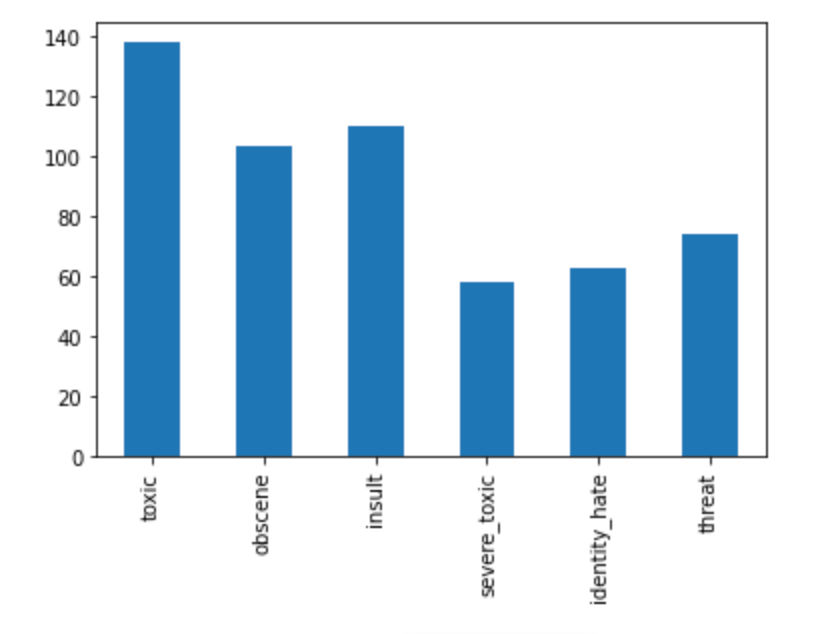Label distribution by sampling examples using conditional probabilities and NNLS, with a target of N = 100 examples for each of the 6 toxic categories.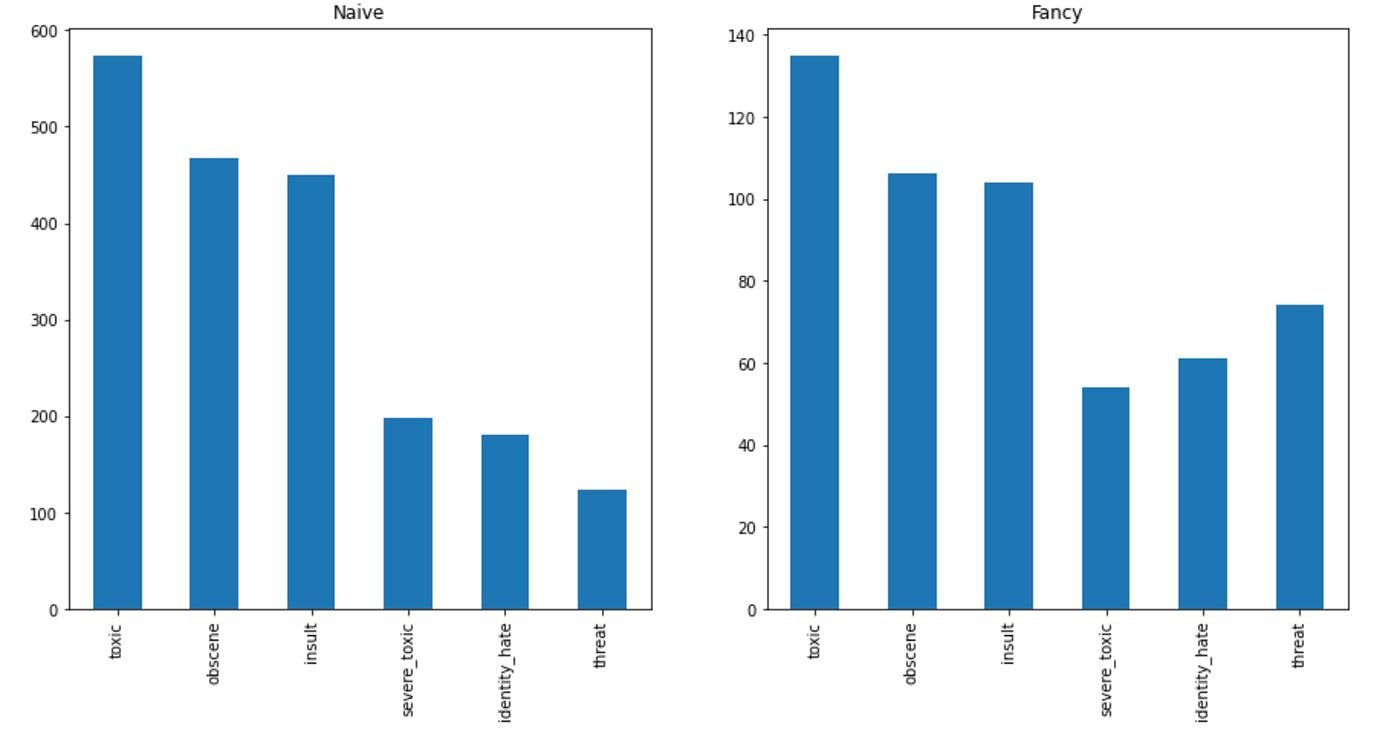Side by side comparison of the naive and fancy sampling method.

It is important to note that this technique can in fact result in fewer than the original target number of examples per target. In the chart above, three of the labels had 60 to 80 examples in the new dataset, when the target was 100. This has an easily fix. The target counts per label that non-linear least squares is optimizing for is arbitrary and any target value can be specified per label — it does not need to be equal across the board. This makes it easy to create a new dataset with a distribution of labels you want, in case you want a new dataset with more examples of a less common label than a common one. It also makes it easy to select for twice as many examples per label as intended, which is an even more enticing solution, given the following fact.

Consider the overall size of the new dataset. If we had used the naive method of sampling, our new dataset would have been made up of ℂ times the number of labels, `C * |labels|`, items in total. With this method, we’re able to have similar label minima, without increasing the total size of the data. In fact, in the sampled dataset above, there are just under 150 items compared to 600 in the naive set! Pragmatically, there is a lot of benefit to this. If we are interested in building a balanced validation set, we retain more items for training. If we want to select items to be annotated, we have fewer in total which means we can annotate more quickly and for a lower price tag overall.

As this is data science, let’s run a quick experiment and make sure we didn’t just get lucky this one time and datasets created by our fancy method are in fact more balanced than the naive method. What we’ll do is create 1,000 different random sampled datasets using the naive method of selecting just based on one label at a time and the fancy method described above using conditional probabilities and non-linear least squares. To compare how balanced each sampled dataset is, we can use a form of categorical entropy for the labels in the dataset. To be clear, this is not cross-entropy which is commonly used as a loss function in trained neural networks. Rather, this is a simple measure of how well balanced the label distribution is in a dataset, based on the probability of a label in the set compared to other labels. Without getting too deep into exactly what entropy is, it represents how surprising on average it is to find a particular label in the dataset.

To calculate this, we calculate the total number of labels across the dataset (this will exceed the total number of items in the set because many items have multiple labels) and find the probability for each label by dividing its count by the total number of labels. The entropy is greatest when every label is equally surprising, i.e., the dataset is perfectly balanced. Therefore, we expect subsets created by the fancy sampling method to have a higher entropy, on average, than those created by the naive sampling technique.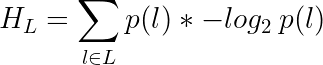Entropy formula for all labels in the set.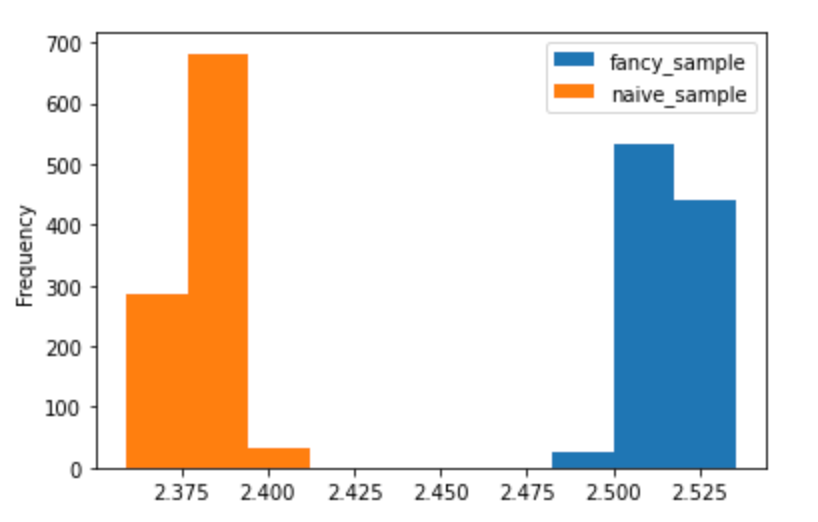Categorical entropy for 1000 subsets created by the naive and by the fancy sampling method.

Wow! A clear winner. Each of the 1000 subsets created by our fancy sampling had a more balanced label distribution than any of those created by naively sampling a fixed number of examples based on a single label. This is great news because it means that the fancy method does, in fact, create balanced sets.

You can find the code for the sampling and visualizations here.

In this blog post, I have presented a way to leverage correlations between labels in a multi-label dataset to create a subset which has a much more balanced distribution of labels. Our team at GumGum uses this method in selecting new data to annotate and re-train our models, so that we don’t spend a lot of time and money annotating web pages that will not improve our models.

Of course, that’s not the only possible use. This could be used to create a validation set that leaves more examples for training. It could also be used to actually intelligently over-sample data and increase the number of examples of less common labels. But, that’s a problem for a future blog post….

Stay tuned for more updates from the GumGum tech teams!

We’re always looking for new talent! View jobs.

--

--

--

## More from GumGum Tech Blog

We’re hiring! Check out https://gumgum.com/engineering

## Most Common PHP Interview Questions & Answers## Top Software Development Trends Of 2021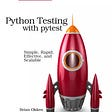## Random Internet Jokes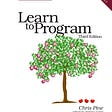## Day 1: Stack On, Stack Off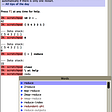## How to deploy your super cool Node app to Azure from GitHub## I wrote a script to get a Banksy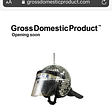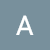## Semantic Label Representation with An Application on Multimodal Product Categorization## Root Cause Analysis in Enterprise Networks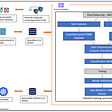## Image Recommendation with Approximate KNN using Collaborative Filtering with ANNoy!## Using graph theory to design experiments